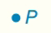Chapter 7.CT, Problem 5CTElementary Geometry For College St...

7th Edition
Alexander + 2 others
ISBN: 9781337614085

Solutions

Chapter
SectionElementary Geometry For College St...

7th Edition
Alexander + 2 others
ISBN: 9781337614085
Textbook Problem

Describe the locus of points in space that are at a distance of 3 cm from point P.To determine

To describe:

The locus of points in space that are at a distance of 3 cm from point P.

Explanation

Definition:

A sphere (the three-dimensional object) is the locus of points in a space that are at a fixed distance from a given point.

The sphere has the fixed point as center, and the fixed distance determines the length of the radius.

Calculation:

A locus the point is the same distance away from the point at all points...

Still sussing out bartleby?

Check out a sample textbook solution.

See a sample solution

The Solution to Your Study Problems

Bartleby provides explanations to thousands of textbook problems written by our experts, many with advanced degrees!

Get Started

What are the domain and range of the function f with the following graph?

Applied Calculus for the Managerial, Life, and Social Sciences: A Brief Approach

Evaluate the definite integrals in Problems 1-32.

Mathematical Applications for the Management, Life, and Social Sciences

Find the limit. 43. limni=1n1n(in)2

Single Variable Calculus: Early Transcendentals

The area of the shaded region is given by:

Study Guide for Stewart's Multivariable Calculus, 8th